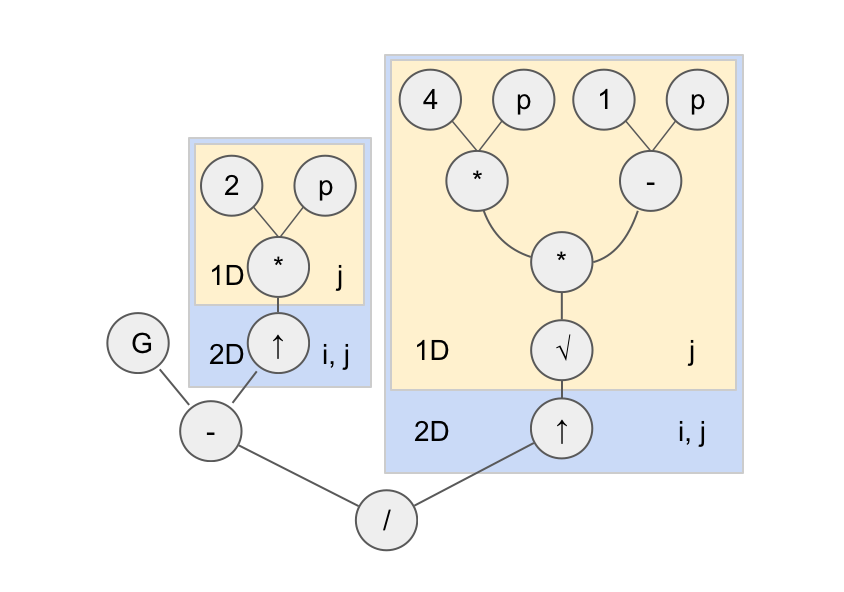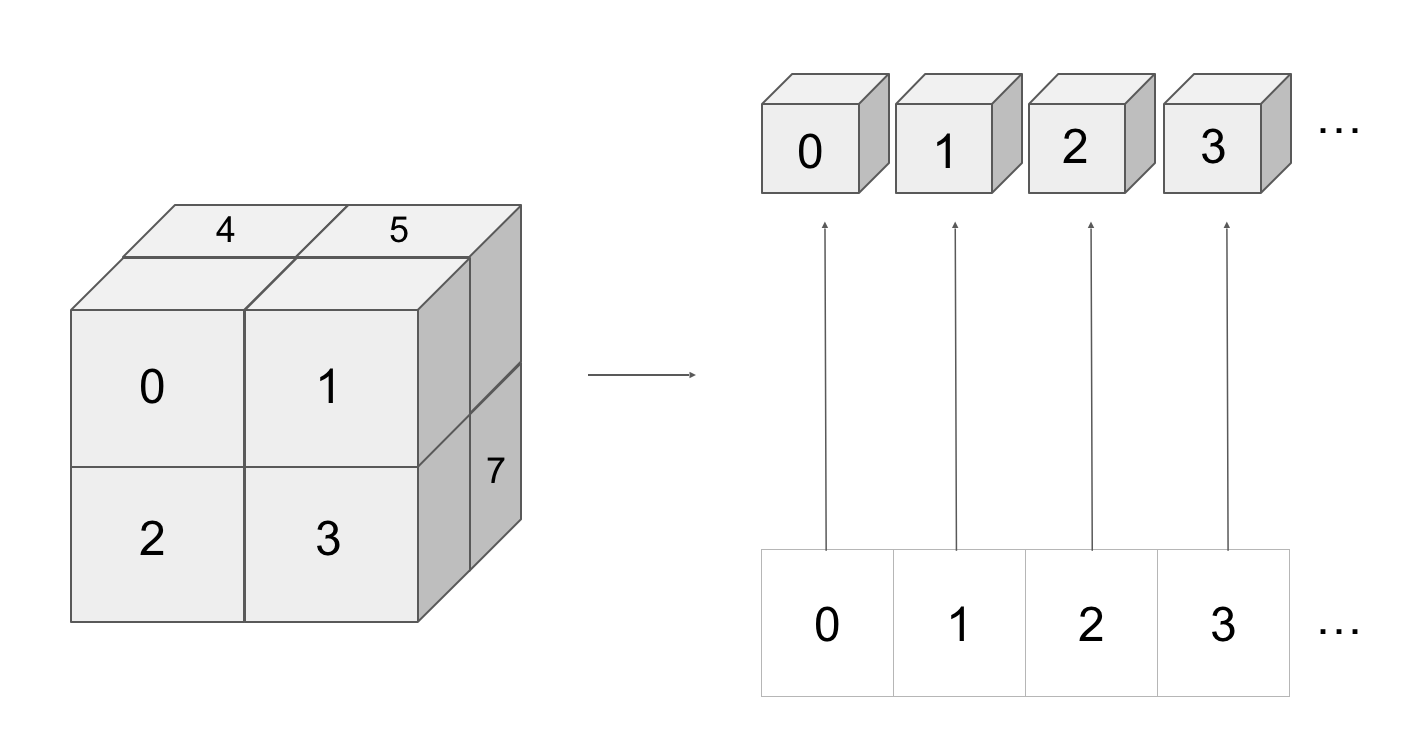# Tensor Algebra in Hail

Instead of taking Spring semester classes, I spent January - June 2019 working as a Software Engineer at the Broad Institute. This work experience was part of Northeastern's co-op program, where students take a break from classes to work a full-time entry-level position in their industry. I spent my six months working on a tool called Hail, a distributed computing engine for doing statistical analyses on big (and I mean very big) genetic data sets.

I spent a large portion of my co-op working on expanding the linear algebra component of hail by implementing the infrastructure necessary in the compiler to represent tensors (arrays, matrices, and higher-dimensional arrays). By being able to express tensors in the language of hail, we could achieve two great feats:

• Users can articulate machine learning models in few lines of code instead of hail engineers writing models on the back-end
• We can optimize across more of a user's pipeline to avoid unnecessary linear algebra computations

My work primarily centered around modeling linear algebra formulas as computation graphs on tensors. When a user writes the following code: (G - 2 * p) / sqrt(4 * p * (1 - p)) in Python, where G is a matrix and p is a vector, instead of directly executing the computation hail constructs a computation graph describing the operations on the data in the equation.Modeling the above equation as this graph allows the compiler to efficiently infer the minimal calculations that must be done to obtain the final result of the equation. It also allows us to save memory and time in minimizing the production of intermediate results, an important optimization on the scale of genetic data.

This model allows us to write down linear algebra in a clear way that runs efficiently. This model also extends to the distributed world, allowing us to consider a very large tensor as a collection of smaller tensors, each on its own machine.The above diagram depicts a distributed 3-tensor, and how it can be represented by blocking multiple local 3-tensors. Extending tensors to be distributed is coming up close in hail's future. Even though my co-op ended and I wasn't around to see this project to completion, I'm greatful for all that I learned about compilers and distributed systems during my co-op. I'm excited to see where hail goes next!